# Tangents from an External Point

Go back to  'Circles-Tangents'

The following figure shows a circle S and a point P external to S. A tangent from P has been drawn to S. This is an example of a tangent from an external point: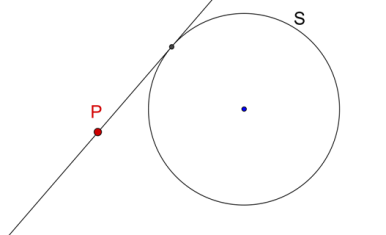How many tangents do you think can be drawn from an external point to a circle? The answer is two, and the following theorem proves this fact.

Theorem: Exactly two tangents can be drawn from an exterior point to a given circle.

Consider the following figure, in which a tangent has been drawn from an exterior point P to a circle S (with center O), and the point of contact is A: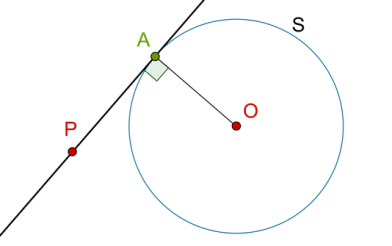We will make use of the fact that $$\angle PAO$$ must be 90 degrees.

Proof: Draw the circle S’ with PO as diameter. Clearly, A must lie on that circle, because we have seen that the angle in a semi-circle is a right angle: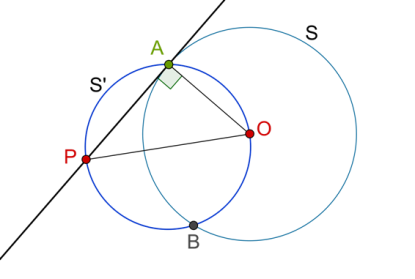We observe that S’ will intersect S in exactly two points, one of which is A, and the other we have labeled as B. The points of contact of the tangents from P to S must be the (two) points of intersection of S’ and S. Thus, exactly two tangents can be drawn from an exterior point to a circle: in this case, PA and PB. The other tangent (with the point of contact being B) has also been shown in the following figure: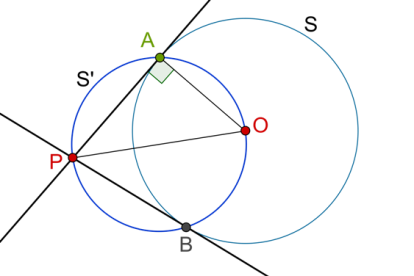We now prove some more properties related to tangents drawn from exterior points.

Theorem: Suppose that two tangents are drawn to a circle S from an exterior point P. Let the points of contact be A and B, as shown: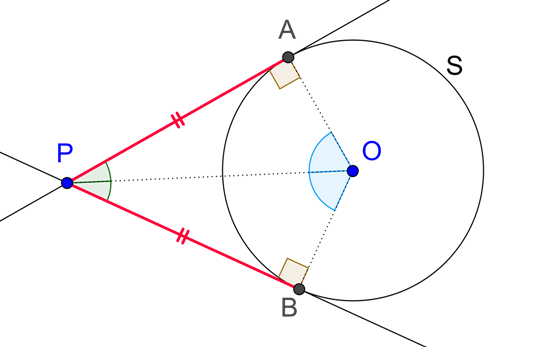Our current theorem says that:

• The lengths of these two tangents will be equal, that is, PA = PB.

• They will subtend equal angles at the center, that is, $$\angle POA{\rm{ }} = \angle POB$$.

• The angle between them will be bisected by the line joining the exterior point and the center, that is, $$\angle APO{\rm{ }} = \angle BPO$$ .

Proof: All the three parts will be proved if we show that $$\Delta PAO$$ is congruent to $$\Delta PBO$$. Comparing the two triangles, we see that:

1. OA = OB (radii of the same circle)

2. OP = OP (common)

3. $$\angle OAP{\rm{ }} = \angle OBP{\rm{ }} = {\rm{ }}{90^0}$$

Thus, by the RHS criterion, $$\Delta {\rm{PAO}}$$ is congruent to $$\Delta {\rm{PBO}}$$, and the truth of all the three assertions follows.

Example 1:  Consider a circle S with center O. From an exterior point, exactly two tangents can be drawn to S. For a point on S, exactly one tangent can be drawn to S. How many tangents can be drawn to S from a point P inside S?

Solution: The answer, of course, is: none. Let us prove this rigorously. If PA is a tangent to S from an internal point P, then the points P, O and A will form a right-angled triangle with hypotenuse OP: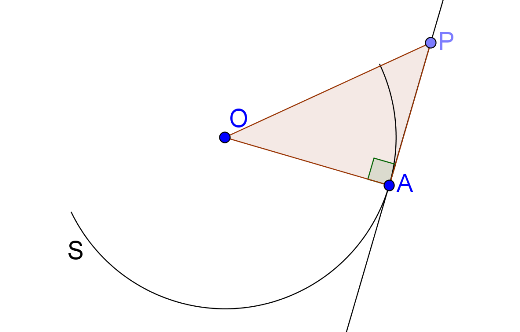Note that OA is a radius of S, whereas since P is inside S, OP must be less than OA. Thus, the triangle above cannot exist. This proves that no tangent can be drawn from an interior point P to S.

You may be saying to yourself: this is so obvious! In fact, I can see it; why do I need to prove it?. The answer to this is that rigor is as important in Mathematics as intuition and visualization, as it leads to (apart from other things) a very good reasoning capability.

Example 2:  Consider the following figure, where BC and BD are tangents to the circle: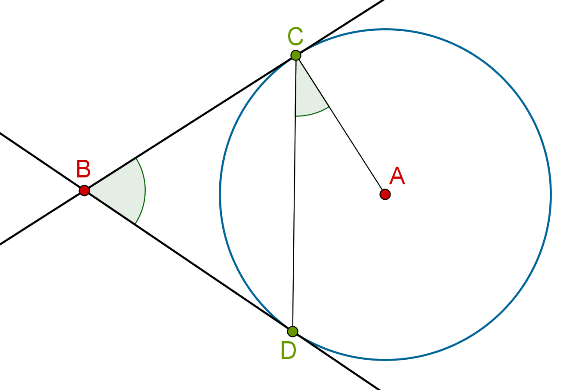What is the relation between $$\angle {\rm{DBC}}$$ and $$\angle {\rm{DCA}}$$ ?

Solution: Since BC is tangent to the circle at C, we note that $$\angle {\rm{BCA }} = {\rm{ }}{90^0}$$ . Thus, $$\angle {\rm{BCD }} = \angle {\rm{BDC }} = {\rm{ }}{90^0}-\angle {\rm{DCA}}$$. Applying the angle sum property in $$\Delta BCD$$, we have:

$$\angle {\rm{DBC }} + \angle {\rm{BCD }} + \angle {\rm{BDC }} = {\rm{ }}{180^0}$$

è$$\angle {\rm{DBC }} + {\rm{ }}\left( {{{90}^0}-\angle {\rm{DCA}}} \right){\rm{ }} + {\rm{ }}\left( {{{90}^0}-\angle {\rm{DCA}}} \right){\rm{ }} = {\rm{ }}{180^0}$$

è $$\angle {\rm{DBC }}-{\rm{ }}2\angle {\rm{DCA }} = {\rm{ }}{0^0}$$

è $$\angle {\rm{DBC }} = {\rm{ }}2\angle {\rm{DCA}}$$

This is the required relation.

Example 3:  From an external point B, tangents BC and BD are drawn to a circle with center A so that the length of each tangent is 4 cm, and AB = 5 cm. What is the radius of the circle?

Solution:  Referring to the figure above for help (even though the length values in it are not the same as in the current problem), note that if AB is joined, then $$\Delta ABC$$ will be right-angled at C, and so the Pythagoras Theorem applies:

$${\rm{A}}{{\rm{B}}^{\rm{2}}} = {\rm{ A}}{{\rm{C}}^{\rm{2}}} + {\rm{ B}}{{\rm{C}}^{\rm{2}}}$$

è $${\rm{A}}{{\rm{C}}^{\rm{2}}} = {\rm{ A}}{{\rm{B}}^{\rm{2}}}-{\rm{ B}}{{\rm{C}}^{\rm{2}}} = {\rm{ }}{{\rm{5}}^{\rm{2}}}-{\rm{ }}{4^2} = {\rm{ }}9$$

è $${\rm{AC }} = {\rm{ }}3{\rm{ }}cm$$

Example 4:  Consider a chord AB of length 9 cm in a circle of radius 5 cm. Tangents at A and B intersect at C, as shown below: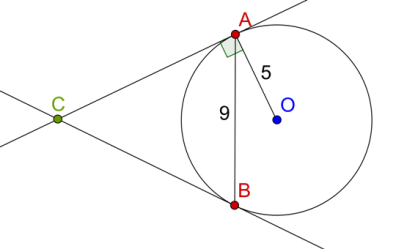What are the lengths of these tangents, that is, of CA and CB?

Solution: Join OC, and let it intersect AB at D: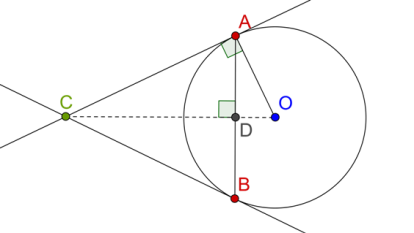Note that $$\angle {\rm{ADC }} = {\rm{ }}{90^0}$$ . Now, compare $$\Delta {\rm{OAC}}$$ with $$\Delta {\rm{ODA}}$$ :

1. $$\angle {\rm{OAC }} = \angle {\rm{ODA }} = {\rm{ }}{90^0}$$

2. $$\angle {\rm{DOA }} = \angle {\rm{COA }}\left( {{\rm{common}}} \right)$$

Thus, the two triangles are similar by the AA similarity criterion. This means that OD:OA = AD:AC (make sure that you understand this). We know that OA = 5 cm, and AD is half of AB, which is 9 cm, so AD is 9/2 cm. We do not know the value of OD, but it can easily be calculated using the Pythagoras Theorem:

$${\rm{O}}{{\rm{D}}^{\rm{2}}} = {\rm{ O}}{{\rm{A}}^{\rm{2}}}-{\rm{ A}}{{\rm{D}}^{\rm{2}}} = {\rm{ }}{5^2}-{\rm{ }}{\left( {9/2} \right)^2} = {\rm{ }}19/4$$

è $${\rm{OD }} = {\rm{ }}\surd \left( {19/4} \right){\rm{ }} = {\rm{ }}\surd \left( {4.75} \right){\rm{ cm}}$$

We plug this value into the similarity relation OD:OA = AD:AC to get:

\begin{align}& {AC = \left( {OA \times AD} \right)/OD}\\& { = \left( {5 \times 9/2} \right)/\surd \left( {4.75} \right) \approx 10.3cm} \end{align}

This is the (approximate) length of the two tangents CA and CB.

Example 5: Consider two concentric circles of radii 3 cm and 5 cm. A chord AB of the larger circle touches the smaller circle at C. What is the length of AB?

Solution: Consider the following figure: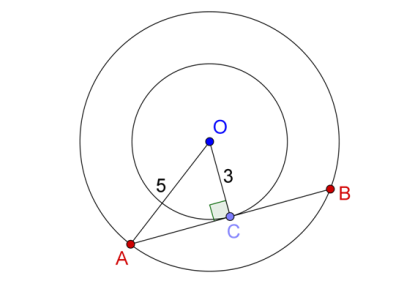Note that since AB is the tangent to the smaller circle at C, OC must be perpendicular to AB. Thus, $$\Delta {\rm{OAC}}$$ is right-angled at C. Also, C must be the midpoint of AB (why?), so $$AC = BC = \raise.5ex\hbox{1}\kern-.1em/ \kern-.15em\lower.25ex\hbox{2} {\rm{ }}AB$$   Using the Pythagoras Theorem, we have:

$${\rm{A}}{{\rm{C}}^2} = {\rm{ O}}{{\rm{A}}^2}-{\rm{ O}}{{\rm{C}}^2} = {\rm{ }}{5^2}-{\rm{ }}{3^2} = {\rm{ }}16$$

è $${\rm{AC }} = {\rm{ }}4{\rm{ }}cm$$

è$${\rm{AB }} = {\rm{ }}2{\rm{ }} \times {\rm{ AC }} = {\rm{ }}8{\rm{ }}cm$$

Example 6: A quadrilateral EFGH circumscribes a circle as shown: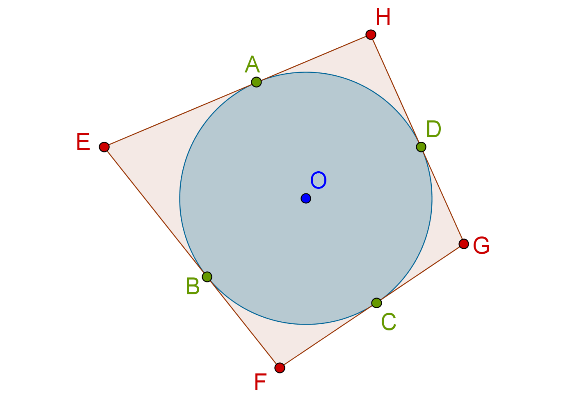Show that EF + GH = EH + FG.

Solution: We use the fact that tangents from an external point to a circle are equal. So, EA = EB, FB = FC, GC = GD and HD = HA, as indicated: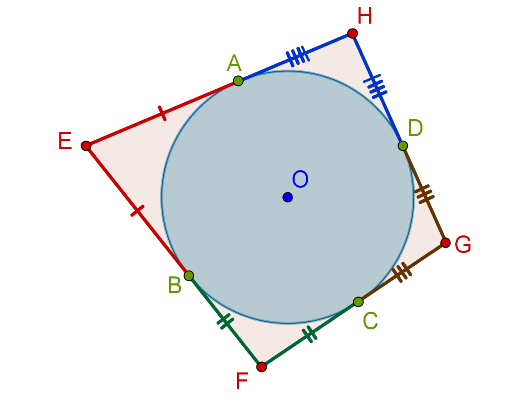Now, it is easy to observe that EF + GH has one part (of each color) from the four sides of the quadrilateral, and similarly, EH + FG has one part of each color from the four sides of the quadrilateral, and thus EF + GH = EH + FG. Make sure that you understand this reasoning; if you have any trouble, write down the values of the sums explicitly.

Example 7: Prove that the opposite sides of a quadrilateral circumscribing a circle subtend supplementary angles at the center of the circle.

Solution: Consider the following figure: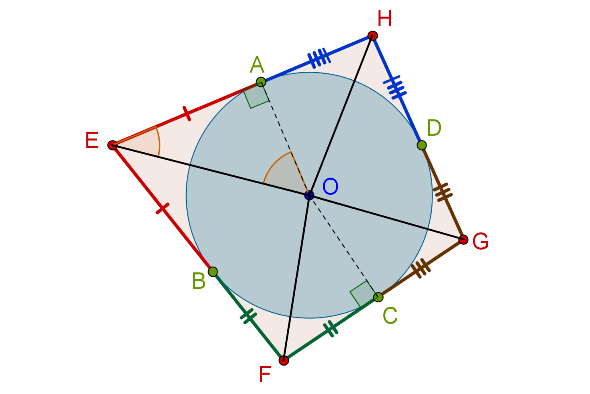Focus your attention on $$\Delta {\rm{AOE}}$$. Since $$\angle {\rm{OAE }} = {\rm{ }}{90^0}$$, we note that $$\angle {\rm{AOE }} + \angle {\rm{AEO }} = {\rm{ }}{90^0}$$. Also, $$\angle {\rm{AEO}}$$ is half of $$\angle {\rm{E}}$$ (can you see why?). Thus,

$\angle AOE{\rm{ }} = {\rm{ }}{90^0}--{\rm{ }}\raise.5ex\hbox{1}\kern-.1em/ \kern-.15em\lower.25ex\hbox{2} \angle E\;\;\;\;\;\;\;\,...\left( 1 \right)$

Similarly, we write the following relations:

\begin{align}& {\angle AOH{\rm{ }} = {\rm{ }}{{90}^0}-{\rm{ }}\raise.5ex\hbox{1}\kern-.1em/ \kern-.15em\lower.25ex\hbox{2} \angle H\;\;\;\;\;\;\;\,...\left( 2 \right)}\\& {\angle COF{\rm{ }} = {\rm{ }}{{90}^0}-{\rm{ }}\raise.5ex\hbox{1}\kern-.1em/ \kern-.15em\lower.25ex\hbox{2} \angle F\;\;\;\;\;\;\;\,...\left( 3 \right)}\\& {\angle COG{\rm{ }} = {\rm{ }}{{90}^0}-{\rm{ }}\raise.5ex\hbox{1}\kern-.1em/ \kern-.15em\lower.25ex\hbox{2} \angle G\;\;\;\;\;\;\;\,...\left( 4 \right)} \end{align}

If we add (1) and (2), we obtain:

$$\angle AOE{\rm{ }} + \angle AOH{\rm{ }} = {\rm{ }}{90^0}-{\rm{ }}\raise.5ex\hbox{1}\kern-.1em/ \kern-.15em\lower.25ex\hbox{2} \angle E{\rm{ }} + {\rm{ }}{90^0}-{\rm{ }}\raise.5ex\hbox{1}\kern-.1em/ \kern-.15em\lower.25ex\hbox{2} \angle H$$

è $$\angle EOH{\rm{ }} = {\rm{ }}{180^0}-{\rm{ }}\raise.5ex\hbox{1}\kern-.1em/ \kern-.15em\lower.25ex\hbox{2} (\angle E{\rm{ }} + \angle H)\;\;\;\;\;\;\;\,...\left( 5 \right)$$

Similarly, adding (3) and (4) will yield:

$\angle FOG{\rm{ }} = {\rm{ }}{180^0}-{\rm{ }}\raise.5ex\hbox{1}\kern-.1em/ \kern-.15em\lower.25ex\hbox{2} \left( {\angle F{\rm{ }} + \angle G} \right)\;\;\;\;\;\;\;\,...\left( 6 \right)$

Finally, if we add (5) and (6) (remember that we are trying to show that $$\angle {\rm{EOH}}$$ and $$\angle {\rm{FOG}}$$ are supplementary), we will have:

$\angle EOH{\rm{ }} + \angle FOG$

$= {\rm{ }}{360^0}-{\rm{ }}\raise.5ex\hbox{1}\kern-.1em/ \kern-.15em\lower.25ex\hbox{2} \left( {\angle E{\rm{ }} + \angle F{\rm{ }} + \angle G{\rm{ }} + \angle H} \right)$

But the sum of the four angles of the quadrilateral must be 3600, and so:

$\angle EOH{\rm{ }} + \angle FOG{\rm{ }} = {\rm{ }}{360^0}-{\rm{ }}\raise.5ex\hbox{1}\kern-.1em/ \kern-.15em\lower.25ex\hbox{2} \left( {{{360}^0}} \right){\rm{ }} = {\rm{ }}{180^0}$

Thus, $$\angle {\rm{EOH}}$$ and $$\angle {\rm{FOG}}$$ are supplementary, and hence, $$\angle {\rm{EOF}}$$ and $$\angle {\rm{GOH}}$$ will also be supplementary.

Circles and Tangents
grade 10 | Questions Set 1
Circles and Tangents
Circles and Tangents
grade 10 | Questions Set 2
Circles and Tangents